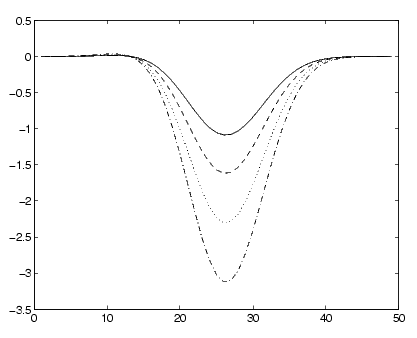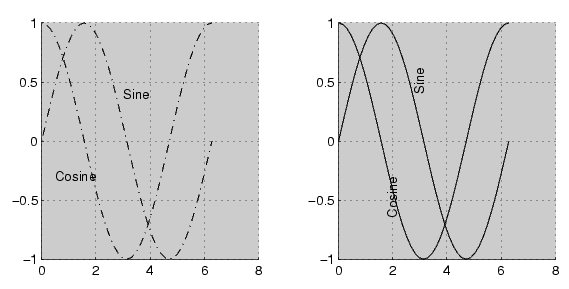GraphicsExamples -- Setting Default Line Styles

The `plot` function cycles through the colors defined by the axes `ColorOrder` property when displaying multiline plots. If you define more than one value for the axes `LineStyleOrder` property, MATLAB increments the line style after each cycle through the colors.

You can set default property values that cause the `plot` function to produce graphs using varying linestyles, but not varying colors. This is useful when you are working on a monochrome display or printing on a black and white printer.

First Example

This example creates a figure with a white plot (axes) background color, then sets default values for axes objects on the root level.

• ```whitebg('w') %create a figure with a white color scheme
set(0,'DefaultAxesColorOrder',[0 0 0],...
'DefaultAxesLineStyleOrder','-|--|:|-.')
```

Whenever you call `plot`,

• ```Z = peaks; plot(1:49,Z(4:7,:))
```

it uses one color for all data plotted because the axes `ColorOrder` contains only one color, but cycles through the linestyles defined for `LineStyleOrder`.Second Example

This example sets default values on more than one level in the hierarchy. These statements create two axes in one figure window, setting default values on the figure level and the axes level.

• ```t = 0:pi/20:2*pi;
s = sin(t);
c = cos(t);
% Set default value for axes Color property
figh = figure('Position',[30 100 800 350],...
'DefaultAxesColor',[.8 .8 .8]);

axh1 = subplot(1,2,1); grid on
% Set default value for line LineStyle property in first axes
set(axh1,'DefaultLineLineStyle','-.')
line('XData',t,'YData',s)
line('XData',t,'YData',c)
text('Position',[3 .4],'String','Sine')
text('Position',[2 -.3],'String','Cosine',...
'HorizontalAlignment','right')

axh2 = subplot(1,2,2); grid on
% Set default value for text Rotation property in second axes
set(axh2,'DefaultTextRotation',90)
line('XData',t,'YData',s)
line('XData',t,'YData',c)
text('Position',[3 .4],'String','Sine')
text('Position',[2 -.3],'String','Cosine',...
'HorizontalAlignment','right')
```

Issuing the same `line` and `text` statements to each subplot region results in a different display, reflecting different default settings.Because the default axes `Color` property is set on the figure level of the hierarchy, MATLAB creates both axes with the specified gray background color.

The axes on the left (subplot region 121) defines a dash-dot line style (-`.`) as the default, so each call to the `line` function uses dash-dot lines. The axes on the right does not define a default line style, so MATLAB uses solid lines (the factory setting for lines).

The axes on the right defines a default text `Rotation` of 90 degrees, which rotates all text by this amount. MATLAB obtains all other property values from their factory settings, which results in nonrotated text on the left.

To install default values whenever you run MATLAB, specify them in your` startup.m` file. Note that MATLAB might install default values for some appearance properties when started by calling the `colordef` command.Defining Default Values Accessing Object Handles© 1994-2005 The MathWorks, Inc.